Electric Field Analogy The electric field is the

• Slides: 22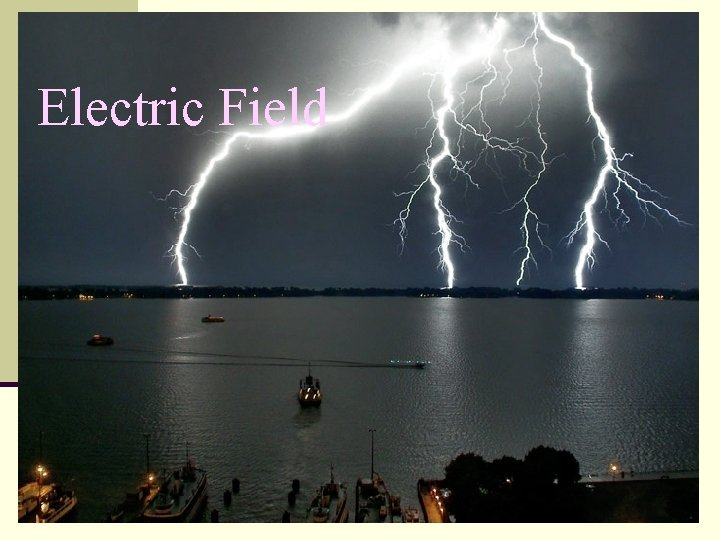Electric Field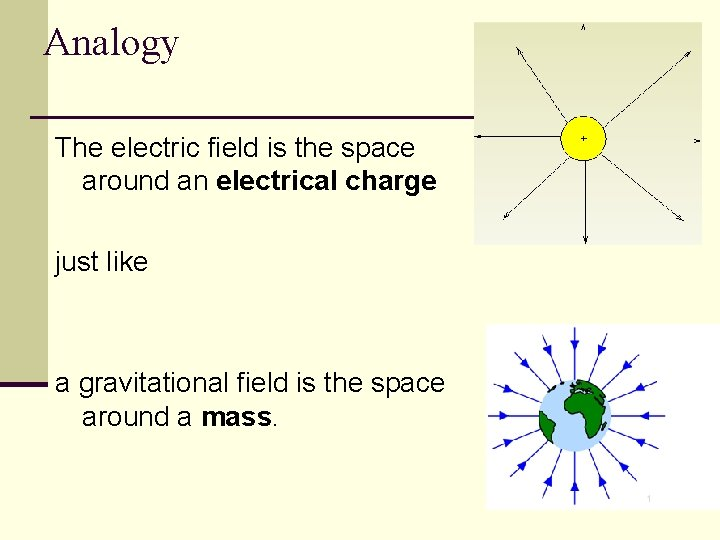Analogy The electric field is the space around an electrical charge just like a gravitational field is the space around a mass.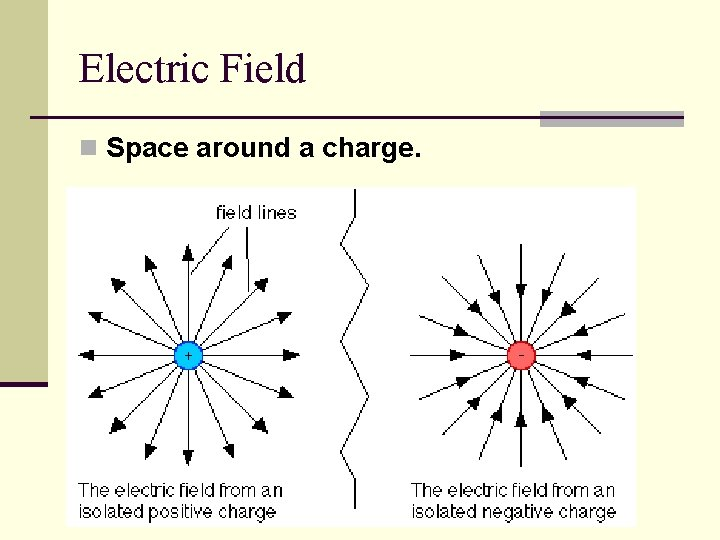Electric Field n Space around a charge.What is the difference?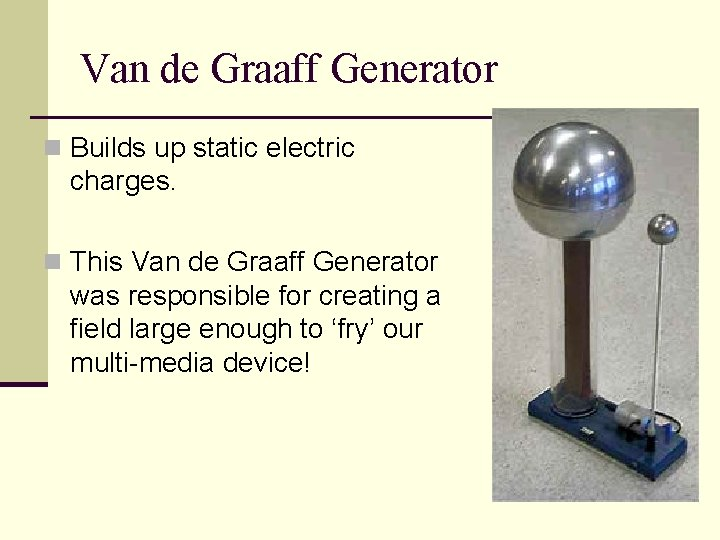Van de Graaff Generator n Builds up static electric charges. n This Van de Graaff Generator was responsible for creating a field large enough to ‘fry’ our multi-media device!Electric Field Vector, E n Electric Field is designed as follows n E = F/ qo n qo , positive test charge n E is a vector quantity n Direction indicated by small + test charge n Unit: N/C n E is analogous to the gravitational field, g, where g=F/mExample 1 n A charge of 3µC is used to test the electric field of a central charge of 6 C that causes a force of 800 N. What is the magnitude of the electric field? n Hint… Which charge ‘tests’ the field n Answer: 2. 7 x 108 N/C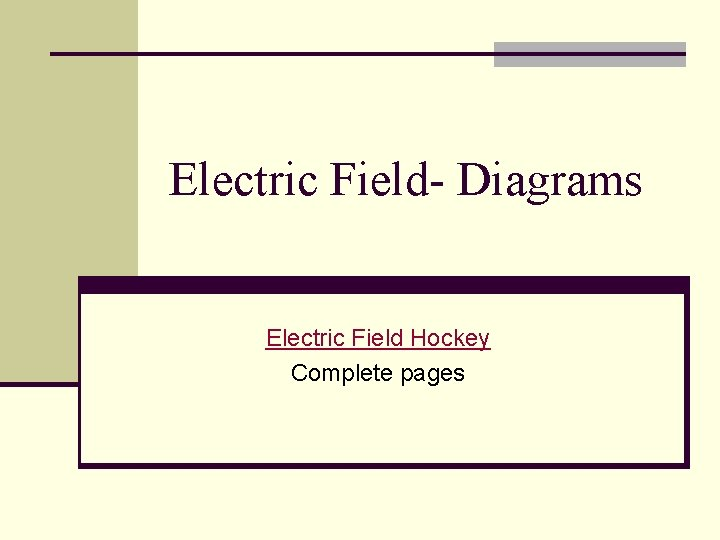Electric Field- Diagrams Electric Field Hockey Complete pages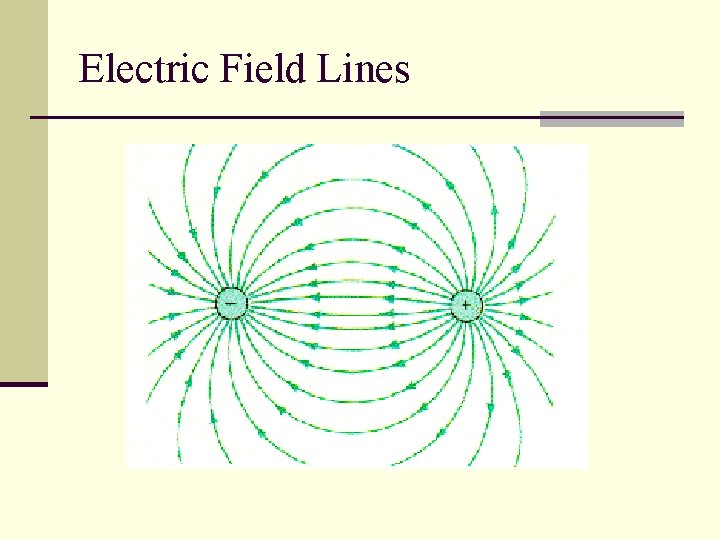Electric Field Lines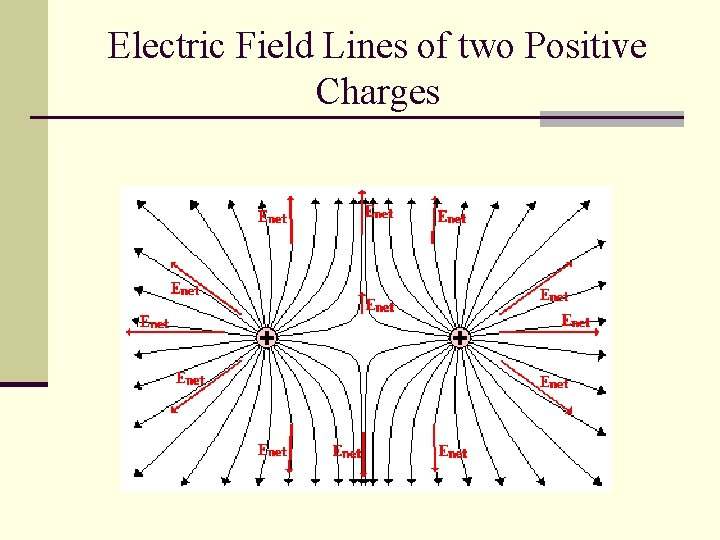Electric Field Lines of two Positive Charges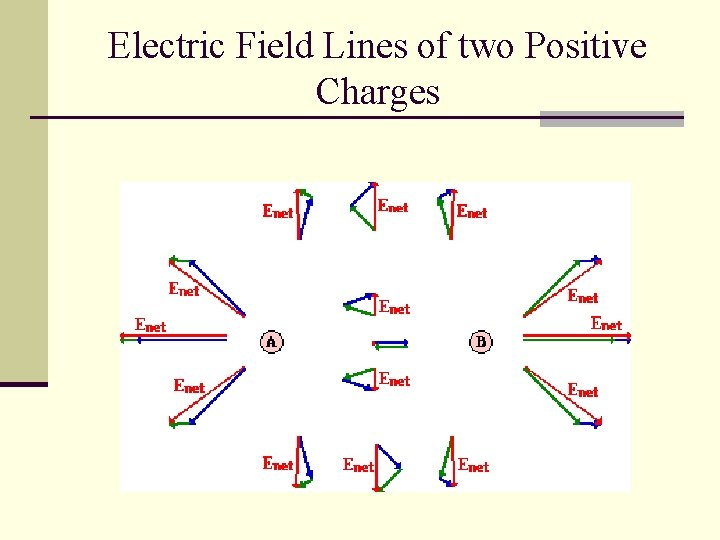Electric Field Lines of two Positive ChargesElectric Field Lines n Lines that indicate the strength and direction of the electric field. n The more dense the lines, the stronger the field. n Electric field vectors are tangent to the curve.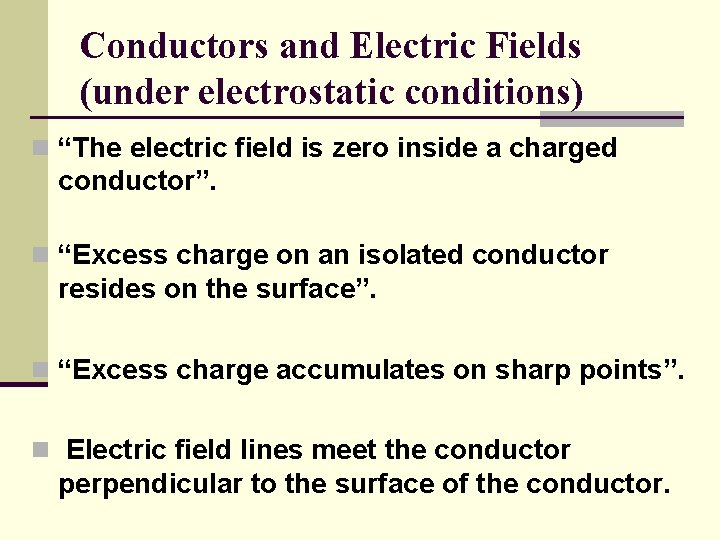Conductors and Electric Fields (under electrostatic conditions) n “The electric field is zero inside a charged conductor”. n “Excess charge on an isolated conductor resides on the surface”. n “Excess charge accumulates on sharp points”. n Electric field lines meet the conductor perpendicular to the surface of the conductor.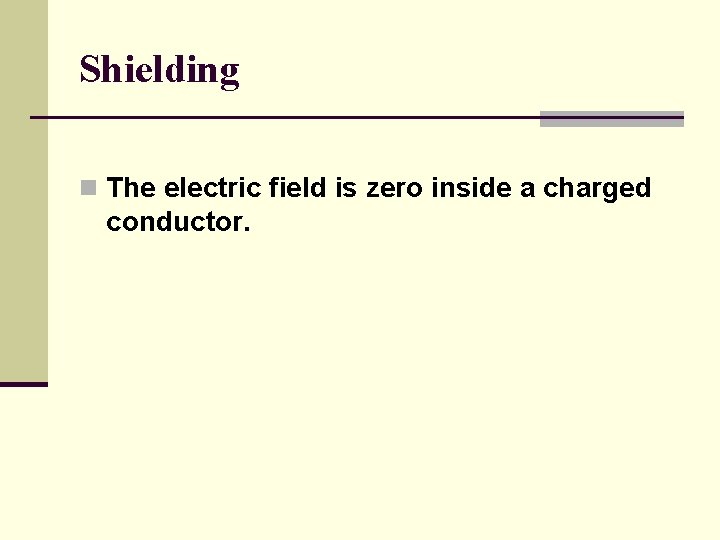Shielding n The electric field is zero inside a charged conductor.Where are you safe during a thunderstorm? A) In a car B) Outdoors or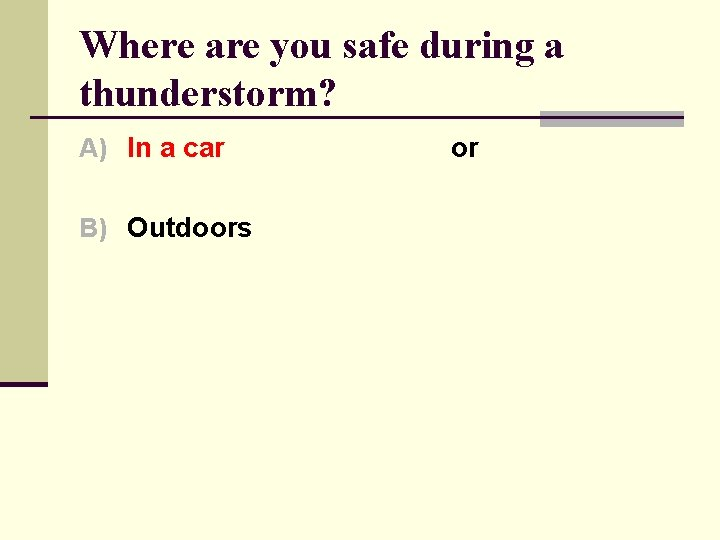Where are you safe during a thunderstorm? A) In a car B) Outdoors or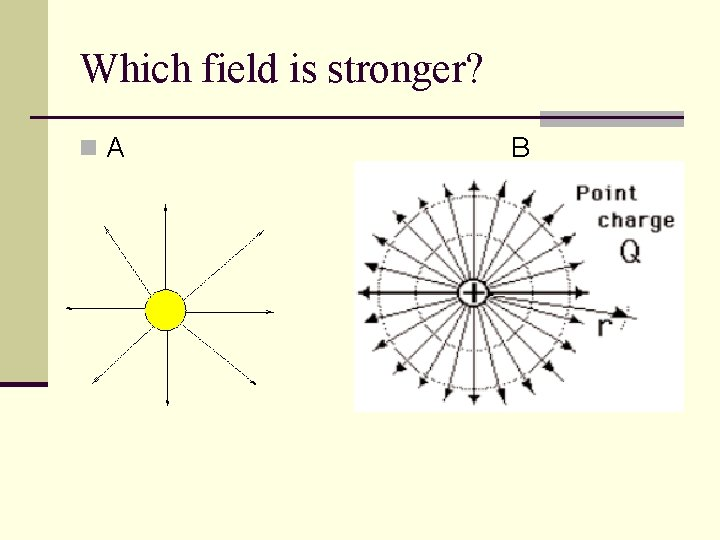Which field is stronger? n. A BWhich field is stronger? n. A B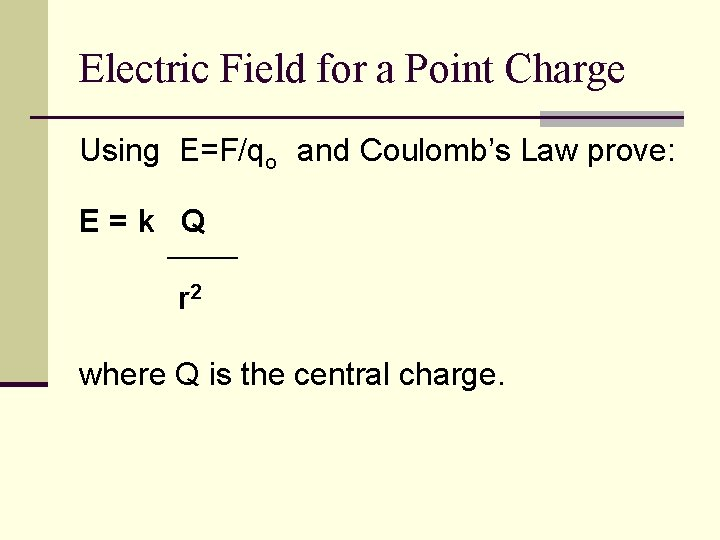Electric Field for a Point Charge Using E=F/qo and Coulomb’s Law prove: E=k Q ______ r 2 where Q is the central charge.Example 2 n A test charge of +3µC is located 5 m to the east of a -4µC charge. n A) Find the electric force felt by the test charge. n B) Find the electric field at that location. n Answer: 4. 32 x 10 -3 N, 1. 44 x 103 N/C along the –x axis.Example 3 n If a test charge is moved to a location three times as far as its original location, how does the electric field change? n Inverse-Square Law says… 1/9Example 4 n Calculate the electric field felt by a positive test charge located half way between a charge of +1 C and a charge of -3 C, that are 2 m apart. n Answer: 3. 6 x 1010 N/C (toward the -3 C charge)